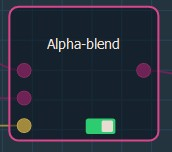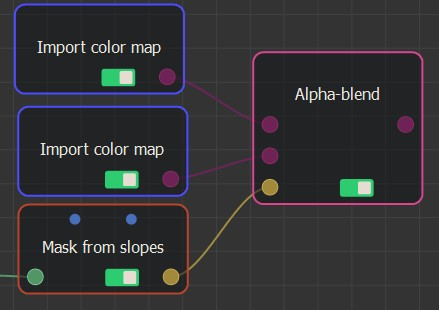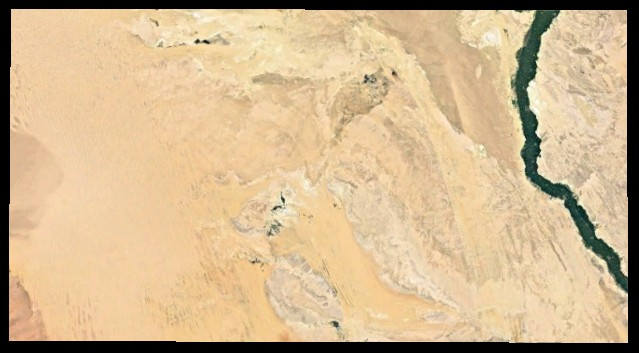### Adding an Alpha blend node

This node creates an alpha composition between two color maps at locations defined by a mask.

The principle of the Alpha blend is as follows:

• In the black areas, the height of the first color map is used.
• In the white areas, the height of the second color map is used.
• Between the two, in the gray areas, the Alpha blend mixes both color maps.

To add a node, right-click in the Graph Editor and select Create Node  > Color management > Alpha blend.This node has no parameters.

Link the Alpha blend node to three input nodes: two color maps and a mask.When you click on the Alpha blend node, the color map is shown as a plane image in the Viewport.At each vertex:

• If the value of the mask is equal to 0 (black areas), the height of the output color map is equal to the height of the first input color map. The second input color map is therefore ignored for this vertex.
• If the value of the mask is equal to 1 (white areas), the height of the output color map is equal to the height of the second input color map . The first input color map is therefore ignored for this vertex.
• If the value of the mask is between 0 and 1 (gray areas), the height of the first input color map is multiplied by the subtraction of 1 and the value of the mask before being added to the height of the second input color map multiplied by the value of the mask. The formula is mask (1 - mask) * color1 + mask * color2.

For example:Both input color maps and the input mask may have different sizes and resolutions. The size and resolution of the output color map is determined by those of the first color map, and the second color map and the mask are enlarged or reduced so that their size corresponds exactly to the size of the output color map.